### Home > A2C > Chapter 1 > Lesson 1.2.1 > Problem1-61

1-61.

For each diagram below, write and solve an equation to find the value of each variable. Give your answer to part (d) in both radical and decimal form. For a reminder of the trigonometry ratios, refer to the Math Notes box for this lesson.

1.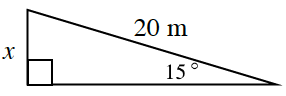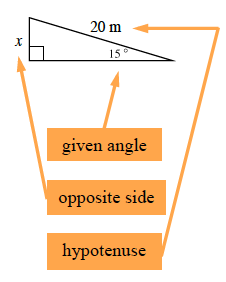$\text{sin}\,\theta=\frac{\text{opposite side}}{\text{hypotenuse}}$

$\text{sin}(15^\circ)=\frac{\textit{x}}{20}$

$20\text{sin}(15^\circ)=20\left(\frac{x}{20}\right)\ \ \ \ \ \ 20\text{sin}(15^\circ)=x$

Use your calculator to compute $x$. Does your answer seem reasonable? If not, verify that your calculator is in degree mode.

1.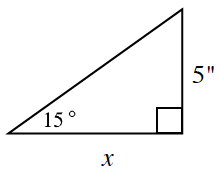$\text{tan}\,\theta=\frac{\text{opposite side}}{\text{adjacent side}}$

Follow the steps in part (a).

1.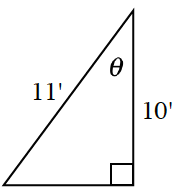$\text{cos}\,\theta=\frac{\text{adjacent side}}{\text{hypotenuse}}$

How can you "undo" cosine?

$\theta=\text{cos}^{-1}\left(\frac{10}{11}\right)\ \ \ \ \theta=24.6^\circ$

1.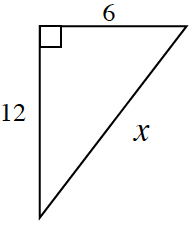An angle is not marked, just three sides. What formula uses three sides of a right triangle?

Pythagorean Theorem